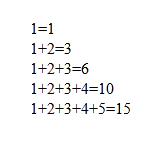# How to add digits of a numeric series

We can print a numeric pyramid series and also can print the sum of every row using php code. We need two ‘for’ loops to print this pattern in php. First ‘for’ loop is used for rows and second ‘for’ loop is used for numbers in a row. We use ‘if’ conditions to add a ‘+’ sign between the numbers also some variables to store the sum of a row.

 ```"; } ?> ```